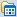# 清静之地

posts - 151, comments - 66, trackbacks - 0, articles - 0
博客园 :: 首页 :: 新随笔 :: 联系 :: 订阅:: 管理### 公告

#include <iostream>
using std::cout;
using std::endl;

int main()
{
const char *pszStr = "this is a string";

// 输出字符串
cout << "字符串：" << pszStr << endl;

// 显然不会输出地址值
cout << "字符串起始地址值： " << pszStr << endl;

return 0;
}


#include <stdio.h>

int main()
{
const char *pszStr = "this is a string";

// 输出字符串
printf("字符串：%s\n", pszStr);

// 输出地址值
printf("字符串起始地址值：%p\n", pszStr);
return 0;
}


#include <iostream>
using std::cout;
using std::endl;

int main()
{
const char *pszStr = "this is a string";

// 输出字符串
cout << "字符串：" << pszStr << endl;

// 如我们所愿，输出地址值
cout << "字符串起始地址值： " << static_cast<const void *>(pszStr) << endl;

return 0;
}